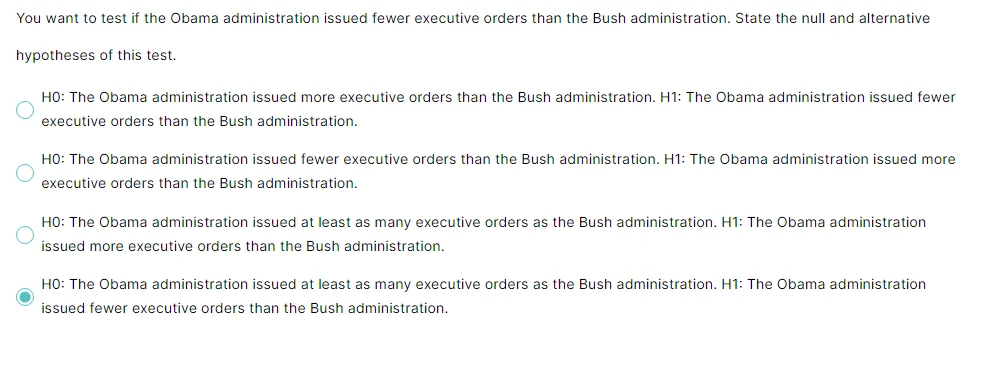04 Aug 2023

Posted on:

08 Jan 2022

1

# can you explain why the last answer is the right one but not the first option???Posted on:

10 May 2022

1

I was wondering the same... Maybe because we are talking about FEWER or less than (<) so we are left with AT LEAST or greater than or equal to (>=). So if you chose answer #1, we would have less than and more than but the 'just as many' would be left untested...

Posted on:

13 Jul 2022

1

I think when we are wording the question with TEST/CHECK, we decide the current status is against what we are testing, so for Obama, we would test/check the statement of fewer orders, which means [H0 the current statute is aginst], and the [H1 else (what we actually test). so, I see (Option#4) is the most suitable answer.

Posted on:

06 Nov 2022

7

The null hypothesis always has the following signs:  =  OR   ≤   OR    ≥

The alternate hypothesis always has the following signs:  ≠   OR  >   OR    <

Example: The average commute time for an ABC employee to and from office is at least 35 minutes.
What will be the null and alternate hypotheses in this case if the average time is represented by μ?
H₀: μ ≥ 35 minutes and H₁: μ < 35 minutes

Posted on:

19 Mar 2023

0

Well, I would like to correct to the others how to chooes the null and alternative based on the question  the null hypothesis always have equal sign (=) thus Obama administration issued fewer executive orders this will be alternative hypothesis because he does not say equal and the null hypothesis will be greater than or equal or at least as many executive orders as the bush administration . the claim here is belong to alternative hypothesis hopfully that help otheres.

Posted on:

04 Aug 2023

0

Hi Asmaa, I did some more research on other websites as I also found it confusing.  In case helpful:

- H0 is the hypothesis we assume to be true before we collect data.  H1 is the hypothesis we want to prove to be true (and therefore dispels or rejects H0)

- H0 usually holds some sort of equals sign eg. =, <=, >=.  It is basically saying 'nothing really changed' as we are at most equal to what we are trying to prove.  H1 holds the opposite sign and is usually eg. Not equals, < or >

- Some examples:

1. The average height of all people in the world is different than 68 inches tall.  Here we are saying height of person < > 68 inches.  This would be alternative hypothesis.

2. Average height of all people in the world is less than 68 inches tall.  Again, using above, this is alternative hypothesis.

3. Average height of all people in the world is less than or equal to 68 inches.  Here we have an equals sign so would be null hypothesis.

4. The impact of Drug A is on average the same as Drug B.  Here we have equals sign so would be null hypothesis.

5. Drug A will on average have a larger impact than Drug B.  This is using only the > sign so would be alternative hypothesis.

Coming back to the Obama question, we want to test if the Obama administration has issued fewer executive orders (so less than) the Bush administration.  Here we are using a < sign.  So it would be the alternative hypothesis with the null hypothesis being Obama has issued at least as many (so <=) as the Bush adminstration.